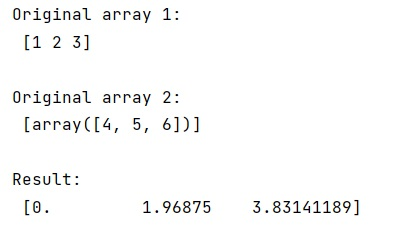# Is it possible to vectorize recursive calculation of a NumPy array where each element depends on the previous one?

Learn, whether it is possible to vectorize recursive calculation of a NumPy array where each element depends on the previous one?
Submitted by Pranit Sharma, on March 22, 2023

Suppose that we are given two numpy vectors (a and b) of the same length and we need to create a new vector c. We need to perform a calculation with i that indicates the element index if we use a loop.

Suppose we need to do something like this:

```c(i) = a(i) + (c(i-1)-a(i))**(-b(i))
```

But it cannot be done in the way it is written above because we can not compute recursion in this way as numpy calculates the whole RHS and then assigns it to the LHS.

Hence, we need to perform this calculation using an explicit loop. We will create an empty array c and assign its values inside a loop.

Let us understand with the help of an example,

## Python code demonstrate whether it is possible to vectorize recursive calculation of a NumPy array where each element depends on the previous one?

```# Import numpy
import numpy as np

# Creating two numpy arrays
a = np.array([1,2,3])
b = np.array([4,5,6])

# Display original arrays
print("Original array 1:\n",a,"\n")
print("Original array 2:\n",[b],"\n")

# Creating an empty array
res = np.empty(3)
res = 0

# Using a loop for giving values to c
for i in range(1,3):
res[i] = a[i] + (res[i-1] - a[i])**(-b[i])

# Display result
print("Result:\n",res,"\n")
```

### Output